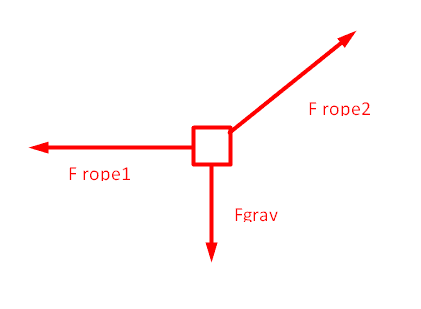# Concept of force components

• StudentElias

## Homework Statement

A box of mass X kg hangs motionless from two ropes, as shown in the diagram. The angle of rope 1 is specified amount of degrees. Choose the box as the system. The x-axis runs to the right, the y-axis runs up, and the z-axis is out of the page.

What is the magnitude of |F2|?

## Homework Equations

[/B]
The equation for finding the magnitude of the force is Force/cos(theta).
The equation for finding the force on the x-axis is |F2|sin(theta).

## The Attempt at a Solution

[/B]
I understand that the formula for |F2| is F2y/cos(theta), but I was wondering what the concept behind it would be? My book describes it as using "directional" cosines? It

Also, for F2x, I'm extremely confused as to why the F2 needs to be multiplied by sin(theta), rather than cosine theta, other than it just giving you F2y. My book doesn't say anything about finding F2x, but is it related to taking the derivative of cosine?
It seems counter-intuitive to use cosine for the y-axis and sine for the x axis, but that's the process to get the same value as the correct answer?

Here's the free body diagram:#### Attachments

Last edited:

To carry out a force balance in the vertical direction, in terms of the magnitude of F1, what is the component of F1 in the vertical direction?

I named the ropes inversely, but F1 would be 0 because it's the flat line. Sorry about the mixup, I'll fix that.
F2 is the opposite of gravity because it cancels out gravity's force, so -mg.

I named the ropes inversely, but F1 would be 0 because it's the flat line. Sorry about the mixup, I'll fix that.
F2 is the opposite of gravity because it cancels out gravity's force, so -mg.
No. F2 is not equal to mg. In terms of the magnitude of F2, what is the vertical component of F2?

I thought the vertical component was the opposite of mg, but is it mg times sin(theta) rather than that?

I thought the vertical component was the opposite of mg, but is it mg times sin(theta) rather than that?
Like I said, in terms of the magnitude of ##F_2##, what is the component of ##F_2## in the vertical direction.

Would it be this?
|F2y| = Fgrav + Ftension
I'm not quite sure what else it could be than that. It seems to be what would be the actual equation for it though. There's just no acceleration in the problem, which would cause it to be canceled out.

Last edited:
Would it be this?
|F2y| = Fgrav + Ftension
I'm not quite sure what else it could be than that..
In terms of the magnitude of F2, the component of F2 in the vertical direction is $$F_{2y}=|F_2|\sin{\theta}$$Does this make sense to you? If so, then, in terms of the magnitude of F2, what is the component of F2 in the horizontal direction?

Oh. For this problem, doing that made my homework site count it wrong for some reason, so I ruled it out.

F2x would equal |F2|cos(theta) based on that.

F2 would be the weight plus any force being exerted that moves it, but it's not moving so it's just the weight?

Oh. For this problem, doing that made my homework site count it wrong for some reason, so I ruled it out.

F2x would equal |F2|cos(theta) based on that.

F2 would be the weight plus any force being exerted that moves it, but it's not moving so it's just the weight?
No. Please write down the force balances in the vertical and horizontal directions in terms of the components of F2 in the horizontal and vertical directions that you just determined.

F2 = <|F2|cos(theta), |F2|sin(theta), 0>
And its magnitude is equal,
|F2| = sqrt((|F2|cos(theta))^2 + (|F2|sin(theta))^2)
= sqrt(|F2|^2 * (cos^2 (theta) + sin^2 (theta)))
= sqrt(|F2|^2)
= |F2|

For some reason |F2| came out equal to F2y/cos(theta) on my homework, but that's saying that |F2| is also equal to |F2|sin(theta)/cos(theta) or |F2|tan(theta)? Is it just coincidence?

F2 = <|F2|cos(theta), |F2|sin(theta), 0>
And its magnitude is equal,
|F2| = sqrt((|F2|cos(theta))^2 + (|F2|sin(theta))^2)
= sqrt(|F2|^2 * (cos^2 (theta) + sin^2 (theta)))
= sqrt(|F2|^2)
= |F2|

For some reason |F2| came out equal to F2y/cos(theta) on my homework, but that's saying that |F2| is also equal to |F2|sin(theta)/cos(theta) or |F2|tan(theta)? Is it just coincidence?
You don't seem to want to answer my question, so I'll answer it myself (again).

FORCE BALANCES
$$|F_2|\sin{\theta}-mg=0$$
$$|F_2|\cos{\theta}-F_1=0$$
Do you agree with these equations? If you solve the first equation for ##|F_2|##, what do you get? If you substitute that result into the 2nd equation and solve for ##F_1##, what do you get?

I didn't quite realize what you meant by force balances, I'm really sorry about that. For some reason I saw "components of F2" and assumed it to be the components in the vector.
It would end up as:
|F2| = mg/sin(theta)

•